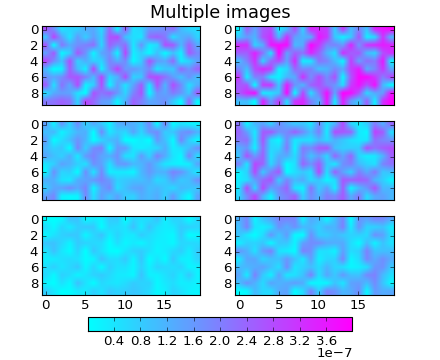# pylab_examples example code: multi_image.py¶```#!/usr/bin/env python
'''
Make a set of images with a single colormap, norm, and colorbar.

It also illustrates colorbar tick labelling with a multiplier.
'''

from matplotlib.pyplot import figure, show, axes, sci
from matplotlib import cm, colors
from matplotlib.font_manager import FontProperties
from numpy import amin, amax, ravel
from numpy.random import rand

Nr = 3
Nc = 2

fig = figure()
cmap = cm.cool

figtitle = 'Multiple images'
t = fig.text(0.5, 0.95, figtitle,
horizontalalignment='center',
fontproperties=FontProperties(size=16))

cax = fig.add_axes([0.2, 0.08, 0.6, 0.04])

w = 0.4
h = 0.22
ax = []
images = []
vmin = 1e40
vmax = -1e40
for i in range(Nr):
for j in range(Nc):
pos = [0.075 + j*1.1*w, 0.18 + i*1.2*h, w, h]
if i > 0:
a.set_xticklabels([])
# Make some fake data with a range that varies
# somewhat from one plot to the next.
data =((1+i+j)/10.0)*rand(10,20)*1e-6
dd = ravel(data)
# Manually find the min and max of all colors for
# use in setting the color scale.
vmin = min(vmin, amin(dd))
vmax = max(vmax, amax(dd))
images.append(a.imshow(data, cmap=cmap))

ax.append(a)

# Set the first image as the master, with all the others
# observing it for changes in cmap or norm.

class ImageFollower:
'update image in response to changes in clim or cmap on another image'
def __init__(self, follower):
self.follower = follower

norm = colors.Normalize(vmin=vmin, vmax=vmax)
for i, im in enumerate(images):
im.set_norm(norm)
if i > 0:
images.callbacksSM.connect('changed', ImageFollower(im))

# The colorbar is also based on this master image.
fig.colorbar(images, cax, orientation='horizontal')

# We need the following only if we want to run this interactively and
# modify the colormap:

axes(ax)     # Return the current axes to the first one,
sci(images)  # because the current image must be in current axes.

show()
```

Keywords: python, matplotlib, pylab, example, codex (see Search examples)